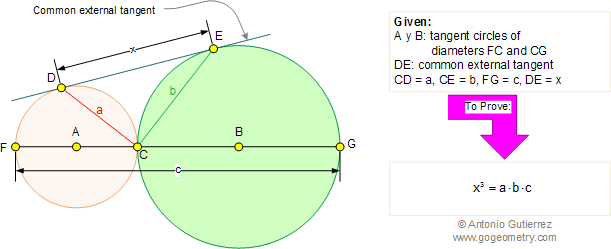Problem 272: Tangent Circles, the Cube of the Common external tangent In the figure below, A and B are tangent circles of diameters FC and CG respectively. If DE is the common external tangent, CD = a, CE = b, FG = c, and DE = x, prove that.Recent Additions